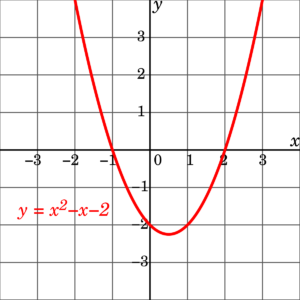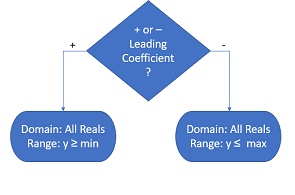# Quadratic Function (Polynomial), Equation & Formula

Contents:

## 1. What is a Quadratic Function?

A quadratic function (also called a quadratic, a quadratic polynomial, or a polynomial of degree 2) is special type of polynomial function where the highest-degree term is second degree.

The general form of a quadratic function is:
f(x) = ax+ bx + c (or y = ax+ bx + c) ,
where a, b and c are all real numbers and a cannot be equal to 0.

The graph of a quadratic function is a parabola, a 2-dimensional curve that looks like either a cup(∪) or a cap(∩).

The quadratic function y = x2 – x – 2 is plotted below:A quadratic polynomial with two real roots (x-axis crossings), which means no complex roots. A quadratic function that has a minimum above the x axis would have no real roots and two complex roots.

The “a” variable of the quadratic function tells you whether a parabola opens up (more formally called concave up) or opens down (called concave down).  In the graph above the variable x is positive so that parabola opens up.  If the variable x were negative, like -3x2, the parabola would open down.  A parabola that opens up has a vertex that is a minimum point.  A parabola that opens down has a vertex that is a maximum point.  Quadratic functions are useful when trying to solve problems involving quantities with unknown variables.

The quadratic function is an example of a second-degree polynomial.  What this means is that the highest degree that a variable in the function can have is 2.  One way to remember this is to keep in mind that the term quadratic comes from the word quadrate which means square (we are looking for a function where squared is the highest variable).

## Examples

• f(x) = x is a quadratic function because the degree of variable x is 2.
• f(x) = 2x+ 5x + 3 is a quadratic because the highest degree of all of the variables is 2.  Note that the degree of variable 5x is 1.
• f(x) = x3 is not a quadratic. The degree of the x variable is 3.  Again, the highest degree of an x variable in a quadratic function is 2.
• f(x) = x5 – 6x2.  This one is a bit more tricky since we have an x variable with a degree of 2.  However, the highest degree in the function is a 5, which violates the general rule above.  Thus, this function is not a quadratic function.

## Domain and Range of a Quadratic Function

A quadratic function is a type of polynomial function. You can use the following flow chart to find the domain and range:Watch this short video on how to find the domain and range of a quadratic function using the flowchart and a graph:

## What is a Quadratic Term?

A quadratic function or equation has the form f(x) = ax2 + bx + c. It contains three terms:

For example, for the function f(x) = 5x2 + 4x – 2, the quadratic term is 5x2. In the expression f(x) = x2 + 2, it’s x2. The sign of the leading coefficient affects the shape of the parabola:

• A positive coefficient will result in a “U” shape,
• A negative coefficient will result in a “∩” shape.

It’s called a quadratic term because it’s the term that makes the expression a quadratic function: take away the “ax2” and you’re left with a linear function, bx + c.

Projectile motion can be described by, in part, a quadratic term. For example, the following graph shows the path of a baseball:The expression needed to fully explain the baseball’s path has three parts:

• The quadratic term represents the ball’s motion due to gravity. That’s what gives the path the distinctive quadratic (i.e. humped) shape: acceleration slows down as gravity pulls on the ball. Eventually the ball reaches its peak height, then gravity slows the ball down further until it eventually hits the ground.
• The linear term represents the vertical (upwards) motion of the ball after it is hit by the bat. This part of the expression would be a straight line, except that gravity bends it downwards.
• The constant term represents the ball’s initial height when hit. For a baseball, this would be roughly 2-3 ft, depending on the height of the player.

In calculus, we’re mostly concerned with functions.

An equation is simply an expression with two equal terms. For example:
y = ax2 + bx + c
If the quadratic equation meets the requirement for functions (that each input is matched to at most one output), then it’s called a quadratic function. Usually, you would give that function a name:
f(x) = ax2 + bx + c.
That naming convention, where “f” is the function relationship, means that it’s easier to set quadratic functions and quadratic equations apart.

A quadratic equation always has two solutions, as it is a second-order polynomial. These two solutions can be both either real numbers or complex numbers.

You can find the roots (or zeros) by factoring or by completing the square. Sometimes it may be practical to graph the equation; Often the easiest way to find the roots is by using the quadratic formula, which, when you plug in the a, b, and c of your particular quadratic equation, will give you the roots.This formula can be derived by completing the square of the generalized equation ax2 + bx + c = 0. Completing the square is where you rewrite a polynomial as a quantity raised to the power of “2”.

Quadratic equations will often come up in algebra, and the quadratic formula is worth memorizing. They also have many applications in geometry, engineering, and biology.

## 3. How to Use the Quadratic Formula in Calculus

The quadratic formula is a way to find the solution for any polynomial in the form ax2 + bx + c = 0. You’ll need to use the quadratic formula to find the solutions for polynomials in many places; for example, you can use solutions for polynomials to find total distance for velocity equations. It works when factoring doesn’t, or when factoring is too complicated. In fact, the formula will always give you a solution, while factoring sometimes works and sometimes doesn’t.

The quadratic formula is stated as:
For any function of the form ax2 + bx + c = 0, the value of x is given by:“a”, “b” and c are just numbers, or numerical coefficients. The formula is derived from completing the square.

Example problem: Solve x2 + 3x + 4 using the quadratic formula.

Step 1: Identify a, b and c in your function.
We know that the quadratic formula is: ax2 + bx + c = 0. So, in this example, a = 1, b = 3 and c = 4. Note that there isn’t a number before x2, so we put a “1.” That’s because 1 * x2 = x2.

Step 3:Use algebra to solve the formula:This further simplifies to -8/2, 2/2, or -4, 1, which is the solution for this particular quadratic equation.

Tip: In order for the formula to work, your function must be in the form ax2 + bx + c = 0. You may need to use a little algebra to get your function in the right form. Note that the “2a” in the denominator is underneath everything , not just the square root. Most mistakes are made by accidentally dropping the square root, putting “a” in the denominator instead of “2a” or dropping the “plus/minus.” Also, when you figure out “b2,” the answer is always going to be positive, even if b has a negative before it. Don’t forget to include signs when working through the equation.

## 4. Parabolic Function Graph

A parabolic function graph is any graph that has a “U” (or upside down “U”) shape. In other words, it’s the graph of a quadratic function.

## Basic Form of the Parabolic Function Graph

A parabolic function graph has the basic form of:

f(x) = c(x – a)2 + b

The constants a, b, and c tell you something about:

• Where the graph is located on the x-y plane,
• How steep the shape is,
• Whether the graph is “right side up” or “upside down.”

## A & B: Location of the Vertex of a Quadratic Function

The vertex is the lowest point on the parabola (or if the parabola is inverted, the highest point). For example, the function f(x) = 5(x – 2)2 + 1 has a vertex at (2, 1).## C: Direction and Steepness

The sign of “c” tells you whether the parabola opens up, or down. The following graph shows the function f(x) = 5(x – 2)2 + 1 (shown in red). Changing the sign of the first term to -5 flips the graph upside down:The value of “c” changes the steepness of the graph. In the example below, the same function is graphed with three different values of c. The further away from zero, the steeper the parabolic function graph:Effect of changing the constant c to 1 (red), 5(green) and 20(blue) on a parabolic function graph for the same function.

To compare the steepness of graphs with different signs for c (e.g. -10 and 4), take the absolute values first. For this example, you’ll be left comparing 10 and 4, so 10 is the steeper graph.

## References

Desmos Calculator.
Columbia University. Precalculus: Nonlinear Functions. Retrieved December 6, 2019 from: http://ci.columbia.edu/ci/premba_test/c0331/s4/s4_3.html